Next: More Late-Breaking News ... Up: Order vs. Algebraic Topology Previous: Truncated Lattices

## Triangulations of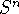In this section we briefly indicate how to construct a large family of examples of finite ordered sets that have a fixed point free automorphism and are such that all retracts have the fixed point property. This shows that despite some nice results for special classes of ordered sets the approach 3 in the introduction might not lead to a resolution of the fixed point problem, as there are too many forbidden retracts. Theorem 4.51 was revealed to the author by an anonymous referee. It also gives a negative answer to Problem 2 in . The author would like to hereby express his gratitude to this referee.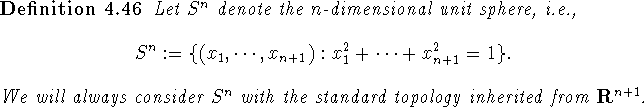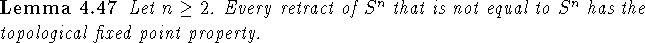Proof: Let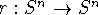be a retraction, i.e., a continuous idempotent map with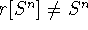. Then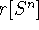is isomorphic to a retract of the n-dimensional unit ball which has the topological fixed point property by Brouwer's fixed point theorem. \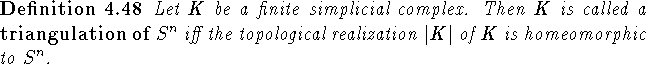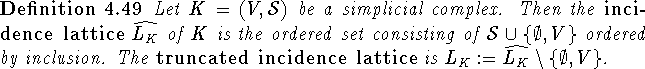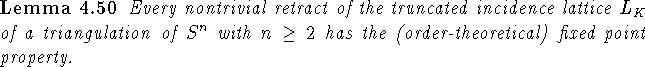Proof: Let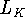be the truncated incidence lattice of the triangulation K of. Let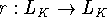be a nontrivial retraction with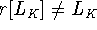. Then there is a minimal element m ofthat is not in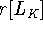: Indeed otherwise r fixes all minimal elements of, which implies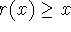for all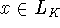. Then there is a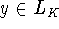such that r(y)>y and r(p)=p for all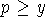. Then y has exactly one upper cover and there is a maximal element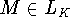such that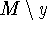has exactly one point (otherwise y has more than one upper cover). Thus M is the only upper bound of y. But then every point x that is in the interior of the topological realization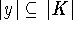of y is such that for all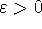small enough we have that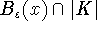is homeomorphic to the upper half space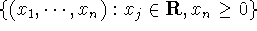. Sinceis an n-dimensional manifold without boundary this is a contradiction to |K| being homeomorphic to.
Let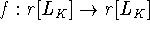be an order-preserving map. Let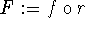. For each minimal element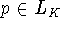choose a minimal element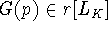such that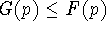. For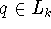not minimal let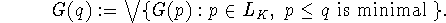Then G is order-preserving on. Moreover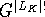is a retraction andinduces a simplicial map on K that is a nontrivial retraction (we have shown above that the retract does not contain all minimal elements), which can be extended to a continuous retraction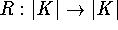. Now by Lemma 4.47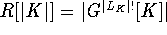has the topological fixed point property. Thus the continuous map on R[|K|] induced by G has a fixed point p. Let S be the smallest simplex in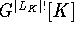such that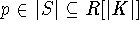. Then G maps S to a sub-simplex of S, i.e.,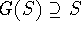and thus G has a fixed point. Thus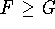has a fixed point, which must be a fixed point of f. \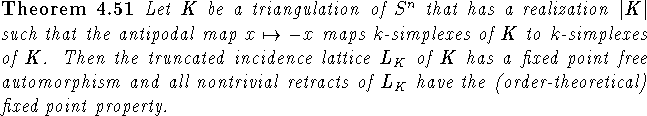Proof: For each simplex S of K let A(S):=-S. By hypothesis this is well-defined. Since no simplex is equal to its antipode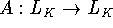is a fixed point free order-preserving automorphism of. By Lemma 4.50 all nontrivial retracts ofhave the (order-theoretical) fixed point property. \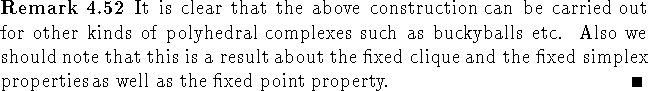Next: More Late-Breaking News ... Up: Order vs. Algebraic Topology Previous: Truncated Lattices

Bernd.S.W.Schroder